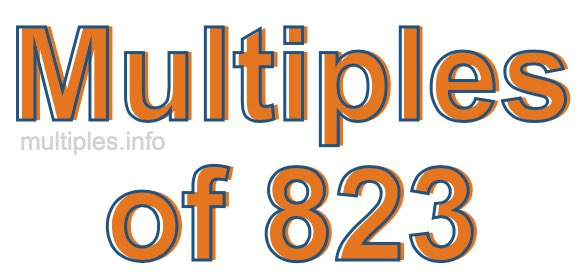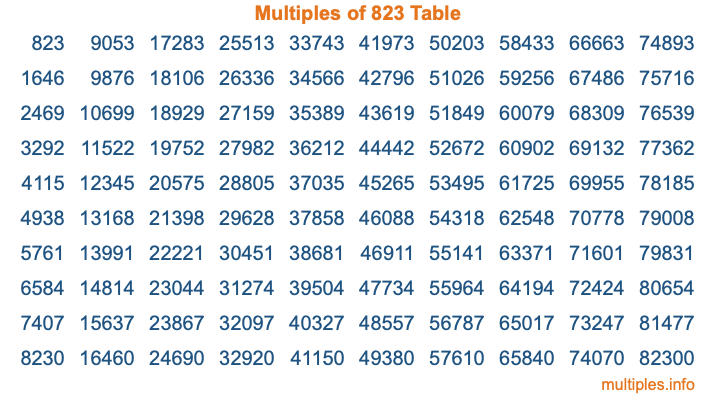Multiples of 823Welcome to the Multiples of 823 page. Here we will first teach you everything you will ever need to know about the multiples of 823, and then give you a study guide summary of everything we taught you to make sure you remember it all. Use this page to look up facts and learn information about the multiples of 823. This page will make you a multiples of eight hundred twenty-three expert!

Definition of Multiples of 823
Multiples of 823 are all the numbers that when divided by 823 equal an integer. Each of the multiples of 823 are called a multiple. A multiple of 823 is created by multiplying 823 by an integer.

Therefore, to create a list of multiples of 823, you start with 1 multiplied by 823, then 2 multiplied by 823, then 3 multiplied by 823, and so on for as long as you want. Thus, the list of the first five multiples of 823 is 823, 1646, 2469, 3292, and 4115. To see a larger list of multiples of 823, see the printable image of Multiples of 823 further down on this page. We also have a category where you can choose any nth multiple of 823.

Multiples of 823 Checker
The Multiples of 823 Checker below checks to see if any number of your choice is a multiple of 823. In other words, it checks to see if there is any number (integer) that when multiplied by 823 will equal your number. To do that, we divide your number by 823. If the the quotient is an integer, then your number is a multiple of 823.

Is  a multiple of 823?

Least Common Multiple of 823 and ...
A Least Common Multiple (LCM) is the lowest multiple that two or more numbers have in common. This is also called the smallest common multiple or lowest common multiple and is useful to know when you are adding our subtracting fractions. Enter one or more numbers below (823 is already entered) to find the LCM.

Check out our LCM Calculator if you need more details about the Least Common Multiple or if you need the LCM for different numbers for adding and subtraction fractions.

nth Multiple of 823
As we stated above, 823 is the first multiple of 823, 1646 is the second multiple of 823, 2469 is the third multiple of 823, and so on. Enter a number below to find the nth multiple of 823.

th multiple of 823

Multiples of 823 vs Factors of 823
823 is a multiple of 823 and a factor of 823, but that is where the similarities end. All postive multiples of 823 are 823 or greater than 823. All positive factors of 823 are 823 or less than 823.

Below is the beginning list of multiples of 823 and the factors of 823 so you can compare:

Multiples of 823: 823, 1646, 2469, 3292, 4115, etc.

Factors of 823: 1, 823

As you can see, the multiples of 823 are all the numbers that you can divide by 823 to get a whole number. The factors of 823, on the other hand, are all the whole numbers that you can multiply by another whole number to get 823.

It's also interesting to note that if a number (x) is a factor of 823, then 823 will also be a multiple of that number (x).

Multiples of 823 vs Divisors of 823
The divisors of 823 are all the integers that 823 can be divided by evenly. Below is a list of the divisors of 823.

Divisors of 823: 1, 823

The interesting thing to note here is that if you take any multiple of 823 and divide it by a divisor of 823, you will see that the quotient is an integer.

Multiples of 823 Table
Below is an image of the first 100 multiples of 823 in a table. The table is in chronological order, column by column. The first column has the first ten multiples of 823, the second column has the next ten multiples of 823, and so on.The Multiples of 823 Table is also referred to as the 823 Times Table or Times Table of 823. You are welcome to print out our table for your studies.

Negative Multiples of 823
Although not often discussed or needed in math, it is worth mentioning that you can make a list of negative multiples of 823 by multiplying 823 by -1, then by -2, then by -3, and so on, to get the following list of negative multiples of 823:

-823, -1646, -2469, -3292, -4115, etc.

Multiples of 823 Summary
Below is a summary of important Multiples of 823 facts that we have discussed on this page. To retain the knowledge on this page, we recommend that you read through the summary and explain to yourself or a study partner why they hold true.

There are an infinite number of multiples of 823.

A multiple of 823 divided by 823 will equal a whole number.

823 divided by a factor of 823 equals a divisor of 823.

The nth multiple of 823 is n times 823.

The largest factor of 823 is equal to the first positive multiple of 823.

823 is a multiple of every factor of 823.

823 is a multiple of 823.

A multiple of 823 divided by a divisor of 823 equals an integer.

823 divided by a divisor of 823 equals a factor of 823.

Any integer times 823 will equal a multiple of 823.

Multiples of a Number
Here you can get the multiples of another number, all with the same attention to detail as we did for multiples of 823 on this page.

Multiples of
Multiples of 824
Did you find our page about multiples of eight hundred twenty-three educational? Do you want more knowledge? Check out the multiples of the next number on our list!